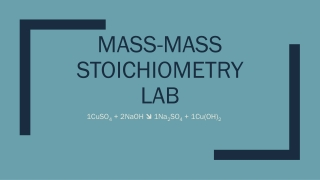DownloadDownload PresentationMass-mass stoichiometry lab

# Mass-mass stoichiometry lab

Télécharger la présentation## Mass-mass stoichiometry lab

- - - - - - - - - - - - - - - - - - - - - - - - - - - E N D - - - - - - - - - - - - - - - - - - - - - - - - - - -
##### Presentation Transcript

1. Mass-massstoichiometrylab 1CuSO4 + 2NaOH  1Na2SO4 + 1Cu(OH)2

2. Why did I have to do stoichiometry calculations? • 0.75 grams of Cu(OH)2 is the amount of product you hoped to form. • In order to do that, we needed to do mass-mass stoichiometry to calculate the amount of BOTH of our reactants that we needed. • You should have calculated 0.61 grams of NaOH and 1.9 grams of CuSO4 • Note: Copper (II) sulfate is sold as a pentahydrate, which means there are 5 waters attached. To calculate the Molar Mass of the compound, you needed to include the 5H2O in your calculation!

3. What is a precipitate? • A precipitate is a solid that is formed from an aqueous (think liquid) solution • The precipitate in our lab was Cu(OH)2 This is indicated with an (s) in the balanced chemical reaction • It should be a pale blue solid, however wet samples of Cu(OH)2 slowly turn black due to the formation of CuO (copper (II) oxide) • What is the clear liquid produced in the lab? • Why did you rinse your product with additional water?

4. Data Table • Mass of NaOH mass that you actually weighed out on the scale of NaOH • Mass of CuSO4· 5H2O  mass that you actually weighed out on the scale of CuSO4· 5H2O • Mass of “marked” filter paper  mass that you weighed out on the scale of your filter paper with your names written on it • Mass of filter paper + dry Cu(OH)2  mass that you weigh out today on the scale of your filter paper with your final product

5. How many grams of copper (II) hydroxide did you actually make in the lab? • You want only the mass of your final product…how do we calculate that? • Mass of filter paper + dry Cu(OH)2 - mass of marked filter paper

6. Stoichiometry Calculations in the Post Lab • Whenever it asks how much product you made in the lab, use stoichiometry with your measured values for your reactants. • Example: if you actually measured 0.63 grams of NaOH, use this value instead of 0.61 grams of NaOH for your calculation. • Why?

7. Percent Error & Sources of Error • Mass of precipitate in lab = mass of Cu(OH)2 you produced (after you subtract off the mass of the filter paper) • Expected mass of precipitate = the mass that we used in our stoichiometry problems for homework (the mass that you wanted to end up with) • If your percent error is higher than 5%, what would some sources of error be? • Mass of Cu(OH)2 is greater than 0.75 grams • Did not wash your product enough  sodium sulfate is still present • Product was not dry  still had water present • Mass of Cu(OH)2 is less than 0.75 grams • Some product was leftover in the beaker and did not make it to the filter paper • Think back to YOUR experiment  is there anything that you did not do completely or fully • Example: CuSO4 or NaOH was not dissolved before you combined them • Hole in your filter paper  was your filtrate (liquid in the E flask tinted blue?)